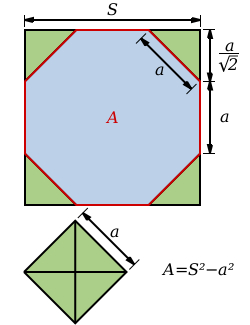A prism

A prism with an altitude of 15cm has a base in the form of a regular octagon inscribed in a square 10cmx10cm. Find the volume of the prism.

V =  1242.6407 cm3

Step-by-step explanation:Did you find an error or inaccuracy? Feel free to write us. Thank you!Tips to related online calculators
Tip: Our volume units converter will help you with the conversion of volume units.
Pythagorean theorem is the base for the right triangle calculator.

You need to know the following knowledge to solve this word math problem:

We encourage you to watch this tutorial video on this math problem: